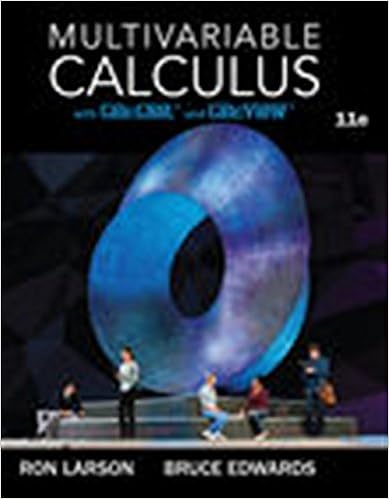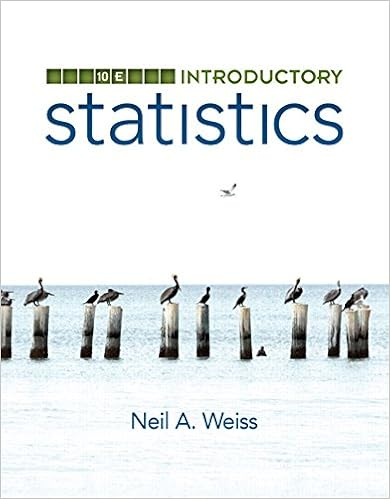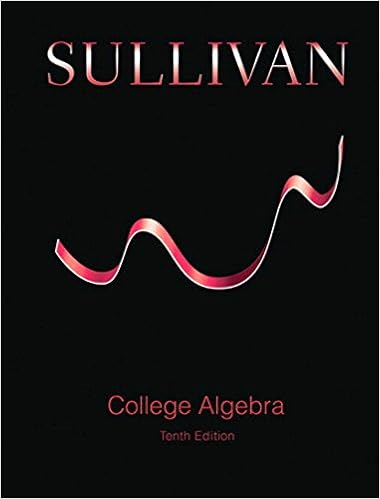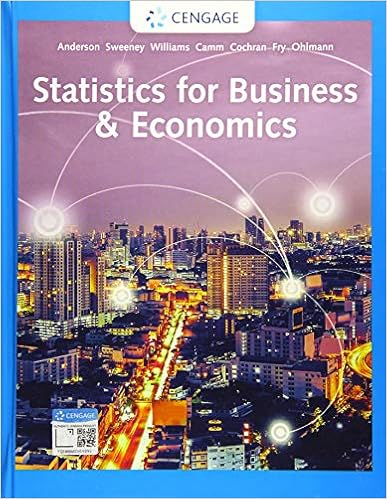# 141BRev - MATH 141B 1. Integration by Parts The formula is:...

• Test Prep
• 14
• 100% (1) 1 out of 1 people found this document helpful

This preview shows page 1 - 3 out of 14 pages.

MATH 141BOverview1. Integration by PartsThe formula is:integraldisplayu dv=uvintegraldisplayv duWe first applied this to the two classes of integrals:integraldisplayxnemxdxandintegraldisplayxnsin(mx)dx,In both cases,nis allowed to be any natural number, andmany real number.The latter class of integrals above works equally well with cos(mx) in place of sin(mx).In each of these cases, letu=xnanddv=emxdxordv= sinmx dx.Integrals from each of these two classes requirenapplications of the integration by parts formula.We also applied the integration by parts formula to the following class of integrals:integraldisplayxnln(mx)dxBothnandmare allowed to be any real number in this case.In this case, letu= ln(mx) anddv=xndx.Integrals in this 3rd class only require only one application of the integration by parts formula.The integration by parts formula gave rise to the important integral (worth remembering).integraldisplaylnx dx=xlnxx2. Improper IntegralsThere are 3 cases here:I.integraldisplayaf(x)dx= limb→∞integraldisplaybaf(x)dxII.integraldisplayb−∞f(x)dx=lima→−∞integraldisplaybaf(x)dxIII.integraldisplay−∞f(x)dx=integraldisplayc−∞f(x)dx+integraldisplaycf(x)dx,for anyc1
##### We have textbook solutions for you!The document you are viewing contains questions related to this textbook.
Chapter 16 / Exercise 12
Multivariable Calculus
LarsonExpert VerifiedBrowse all Textbook Solutions
If the limit defining these integrals exists, then the improper integral is said toconverge. Otherwise,the integraldiverges.Case III reduces the integralintegraltext−∞f(x)dxto an integral of of type I and an integral of type II.In case III, pickc= 0 unless there’s a discontinuity there. First computeintegraltext0f(x)dx. If it diverges,then there is no need to compute the other integralintegraltext0−∞f(x)dx. You simply stop declare that theentire integralintegraltext−∞f(x)dxdiverges.There are 3 ways that an improper integral can diverge. The integral may diverge to +,−∞or itmay be that it diverges, but neither to +nor to−∞.This happens, for instance, when you end upfacing a limit of an oscillating trigonometric function like limx→∞sinx, which does not exist (DNE).One important class of limits we discussed in the course of computing improper integrals was:limn→∞lnxxn= 0,for anyn >0.This expresses the fact that the logarithm grows more slowly than any power ofx. This fact is obviousfor powersn1 (just look at a graph), but for powersnsatisfying 0< n <1,it’s also true.3. Gaussian EliminationRegarding the solution set of any system of linear equations, there are only 3 possibilies:i) There is exactly one solution.ii) There are no solutions.iii) There are infinitely many solutions.The method of Gaussian elimination uses 3 so-calledelementary row operationsto reduce a matrixuntil it satisfies the following conditions:i) All zero rows are grouped at the bottom of the matrix.

Course Hero member to access this document

Course Hero member to access this document

End of preview. Want to read all 14 pages?

Course Hero member to access this document

Term
Spring
Professor
MARCFABBRI
Tags
level curve
##### We have textbook solutions for you!
The document you are viewing contains questions related to this textbook.The document you are viewing contains questions related to this textbook.
Chapter 16 / Exercise 12
Multivariable Calculus
LarsonExpert Verified
•••